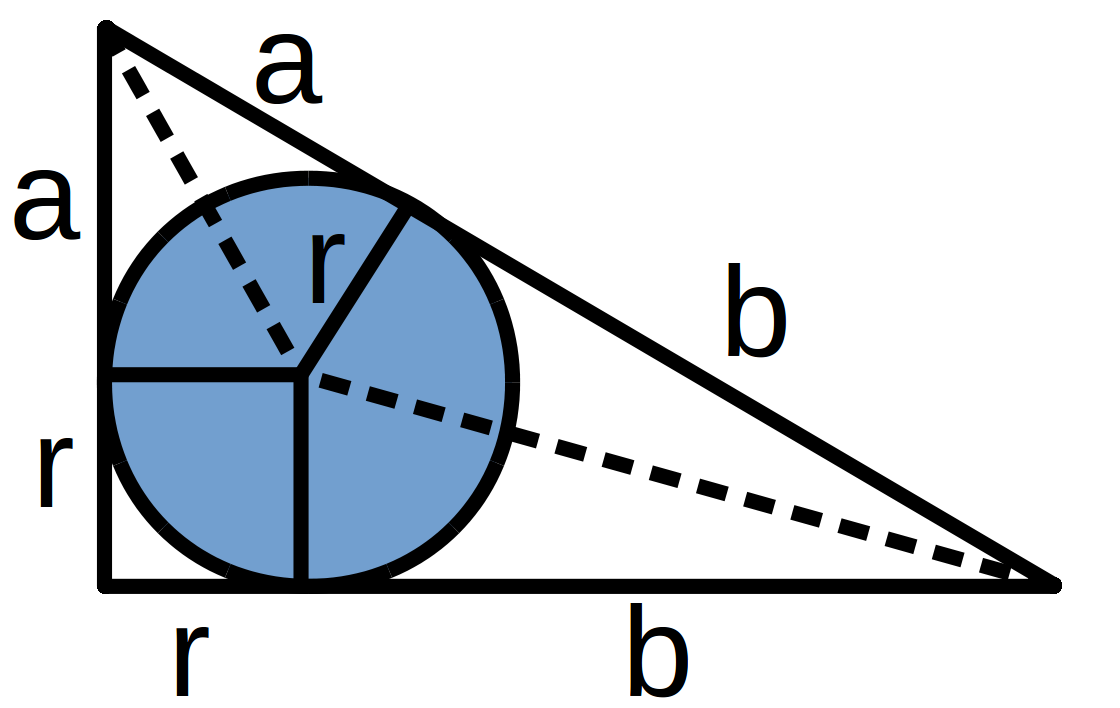# Pythagoras By Incircle

 Recent changes Table of contents
 Links to this page FRONT PAGE / INDEXSubscribe!My latest posts can be found here:
Previous blog posts:
Additionally, some earlier writings:

## 2016/06/08 ...

Some time ago I was working on a puzzle about incircles, and unexpectedly a proof of Pythagoras' Theorem dropped out! I'm sure it's well known to people who know lots about Pythagoras' Theorem, but I thought I'd share it.So take a right-angled triangle, and inscribe an incircle. Suppose the circle is of radius $r$, and the point of contact with the hypotenuse divides it into lengths $a$ and $b.$ Then we have the shorter sides as $a+r$ and $b+r,$ as shown.

Let's think about the area of the triangle. On the one hand it's $\frac{1}{2}(a+r)(b+r),$ but on the other hand it's the square plus each kite, which is $r^2+ar+br.$ That is not instantly obvious, but is left as an exercise for the interested reader. It might help to remember that the centre of the incircle lies on the angle bisectors.

Equating these two expressions for the area we get

$\frac{1}{2}(a+r)(b+r)~=~ar+br+r^2$

Multiply through by 2, expand and simplify:

$ab+ar+br+r^2~=~2(ar+br+r^2)$

$ab~=~r^2+ar+br$

So now let's ask about the square on the hypotenuse.

$(a+b)^2~=~a^2+2ab+b^2$

Substituting in the value for $ab$ we get:

$(a+b)^2~=~a^2+2(r^2+ar+br)+b^2$

Rearrange:

$(a+b)^2~=~(a^2+2ar+r^2)~+~(b^2+2br+r^2)$

So:

$(a+b)^2~=~(a+r)^2~+~(b+r)^2$

And we're done.

 <<<< Prev <<<< A Puzzle About Puzzles : >>>> Next >>>> Representatives Matter ...

 You should follow me on twitter## Comments

I've decided no longer to include comments directly via the Disqus (or any other) system. Instead, I'd be more than delighted to get emails from people who wish to make comments or engage in discussion. Comments will then be integrated into the page as and when they are appropriate.

If the number of emails/comments gets too large to handle then I might return to a semi-automated system. That's looking increasingly unlikely.

# Links on this page

 Site hosted by Colin and Rachel Wright: Maths, Design, Juggling, Computing, Embroidery, Proof-reading, and other clever stuff.Suggest a change ( <-- What does this mean?) / Send me email
Front Page / All pages by date / Site overview / Top of page